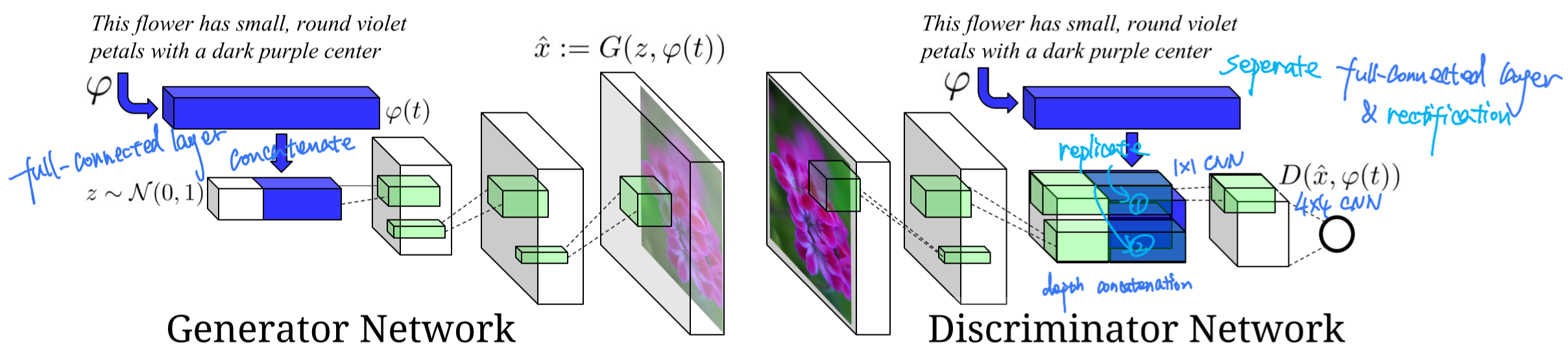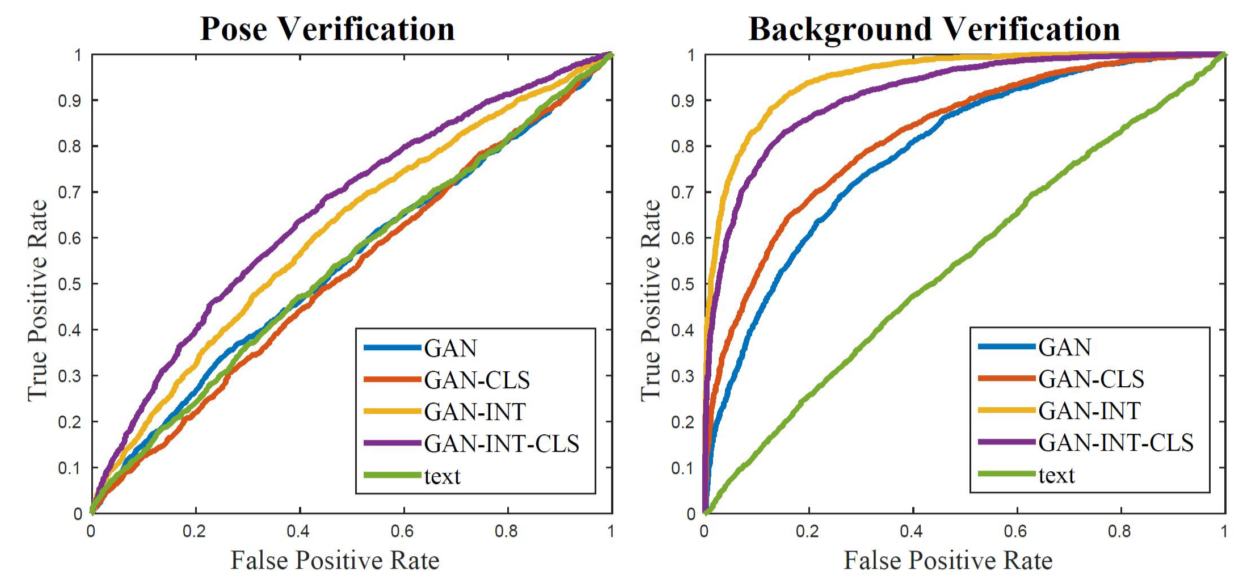# Generative Adversarial Text to Image Synthesis

Modified:

## Overview

For text to image, there are many plausible value to one pixel that correctly illustrate the description of the text, since the the distribution of image conditioned on the text is highly multimodal, e.g. small changes of text description may affect pixel values with low correlation across a wide range.

For image to text, it is much practical to decompose the sequence according to the chain rule to generate captions. TOREAD

## Preliminaries

### GAN

It is common to maximize $\log (D(G(z)))$ for the generator, while it is found to be more effective to minimize maximize $\log (1-D(G(z)))$ instead.

\begin{aligned} \min _{G} \max _{D} V(D, G)=& \mathbb{E}_{x \sim p_{\text { data }}(x)}[\log D(x)]+\\ & \mathbb{E}_{x \sim p_{z}(z)}[\log (1-D(G(z)))] \end{aligned}

### Joint embedding with symmetric structure

The purpose of this structure is to obtain $\varphi(t)$ which can encode text description to visually-discriminative vector.

Denote the classifier as followed, where $\phi(v)$ is the image encoder, and $\varphi(t)$ is the text encoder. Inner-product of vectors can be interpreted to some degree as the distance or similarity of two vectors.

$f_{v}(v) =\underset{y \in \mathcal{Y}}{\arg \max } \mathbb{E}_{t \sim \mathcal{T}(y)}\left[\phi(v)^{T} \varphi(t)\right]$

$f_{t}(t) =\underset{y \in \mathcal{Y}}{\arg \max } \mathbb{E}_{v \sim \mathcal{V}(y)}\left[\phi(v)^{T} \varphi(t)\right]$

Then optimize the following structured loss:

$\frac{1}{N} \sum_{n=1}^{N} \Delta\left(y_{n}, f_{v}\left(v_{n}\right)\right)+\Delta\left(y_{n}, f_{t}\left(t_{n}\right)\right)$

The intuition here is that a text encoding should have a higher compatibility score with image of the corresponding class and vice-versa TOREAD

## Network structure

There is no particular to the total architecture of network, except for the conditions which is encoded from the text description and concatenated to noise afterwards. Two different full-connected layers are implemented to encode text description both in upsampling and downsampling model.### GAN-CLS

Two sources of error should be considered: unrealistic (bad generation) images, and realistic image yet dismatched with the text.

For this reason, apart from origin losses: real image with right text and fake image with false text, additional loss: real image with false text, is added to train the discriminator to have the ability of discriminate whether the generated image match the text or not, instead of only to measure the quality of output image.

\begin{aligned} s_{r} \leftarrow & D(x, h)\{\text { real image, right text }\} \\ s_{w} \leftarrow & D(x, \hat{h})\{\text { real image, wrong text }\} \\ s_{f} \leftarrow & D(\hat{x}, h)\{\text { fake image, right text }\} \\ \mathcal{L}_{D} \leftarrow & \log \left(s_{r}\right)+\left(\log \left(1-s_{w}\right)+\log \left(1-s_{f}\right)\right) / 2 \end{aligned}

### GAN_INT

Deep networks have been shown to learn representations in which interpolations between embedding pairs tend to be near the data manifold (Bengio et al., 2013) TOREAD

Then laryge amount of additional text embeddings can be generated by simply interpolation between captions of training samples.

The generator can learn an ability of generate the ‘interpolated image’ and fill in gaps on the data manifold between training points TOREAD, while discriminator can learn to predict whether image and text pairs match or not.

Note that there is no need to add label of interpolated vector for they are synthetic and fake.

$\mathbb{E}_{t_{1}, t_{2} \sim p_{d a t a}}\left[\log \left(1-D\left(G\left(z, \beta t_{1}+(1-\beta) t_{2}\right)\right)\right)\right]$

### Disentangling style and content

It is a validation method to qualitify the ability of each proposed model to use the noise as style information, in other words, to determine the strength of the effect of noise z in different training methods

By content, we mean the visual attributes of the bird itself, such as shape, size and color of each body part. By style, we mean all of the other factors of variation in the image such as background color and the pose orientation of the bird.

Therefore, generative model must learn to use noise, the prior-condition, to represent style variations in order to generate realistic image.

Inverting generative model $S$ for style transfer is designed to transfer the generative image back into noise z with the following loss,

$\mathcal{L}_{\text {style}}=\mathbb{E}_{t, z \sim \mathcal{N}(0,1)}\|z-S(G(z, \varphi(t)))\|_{2}^{2}$

It is a bit difficult to understand the implication this model. To make sense of this, we can interpret this inverted model $S$ as to measure the ability of $G$ to output a generative image with both decent content and style in convergence case where $\mathcal{L}_{\text {style}}$ comes close to 0.For evaluation, cosine similarity and ROC is used as showed in the above figure. Images with similar and dissimilar content and style is selected and constructed as a pair, and if GAN has disentangled ability, the similarity between noises inverted from images of the same style (e.g. similar pose) should be higher than that of different styles (e.g. different pose).

Tags: